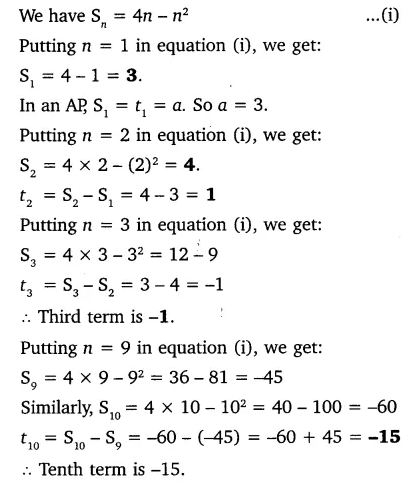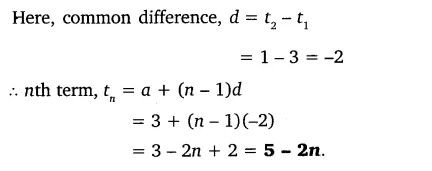# If the sum of the first n terms of an AP is 4n – n2, what is the first term (that is S1)?

If the sum of the first n terms of an AP is 4n – n2, what is the first term (that is S1)? What is the sum of first two terms? What is the second term? Similarly, find the 3rd, the 10th and the nth terms.

1 Like